# Bar Graphs

Go back to  'Data, Statistics'

## Introduction to Bar Graphs

A step ahead from the conventional pictographs, bar graphs are the expected evolution to the visual aids that use bars or rectangular blocks to represent data. Let’s take a look at what makes them so important.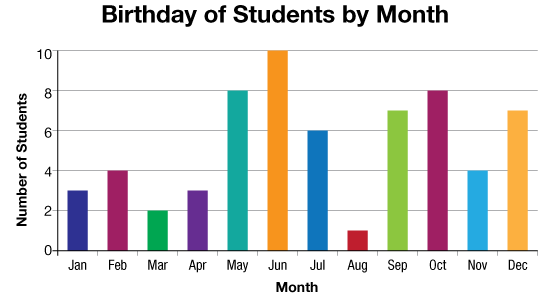## The Big Idea: What are bar graphs?

A bar graph is a specific way of representing categorical data using rectangular bars where the length of each bar is proportional to the value they represent

Bar graphs are an excellent tool when representing data that are independent of one another and don’t need to be in any specific order while being represented.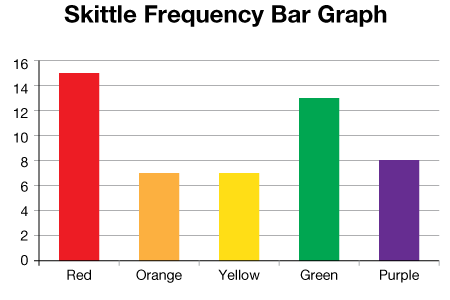Scaled bar graphs, just like scaled pictographs are used to represent large sums of data, scaled down to fit numbers that are easier to work with. Usually this involves the same scaling factor to every entry in a particular graph, that is simply multiplied to the result to get the final number.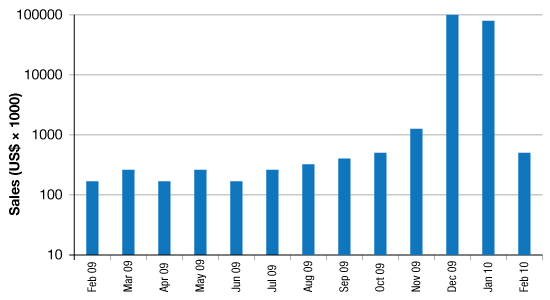To further take up the bar chart example to advanced cases, you would arrive at the double bar graph case where each category is further subdivided into two distinct subclasses that is compared for all various categories.

One example to illustrate is by taking the case of a bar chart that represents the average test score performances for students in a course from different years. The numbers secured by the students each year. Now if you further specify the statistics for the average scores for the boys and girls in each batch, you would end up with a double bar graph.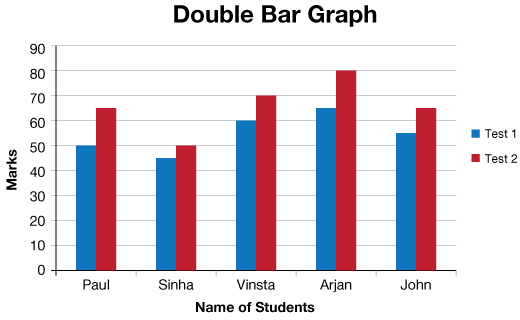This should lend some idea as to the kind of data that bar graphs help elucidate. Discrete intervals with gaps are usually used on the horizontal axis to compare and analyse the values for different categories.

## Why bar graphs? Why are bar graphs important?

The importance of bar graphs come out when we want to compare data sets that are independent from one another. For example, sales report graphs for different quarters or years.

They help in analysing patterns over long periods of time and lend great value to predictive studies.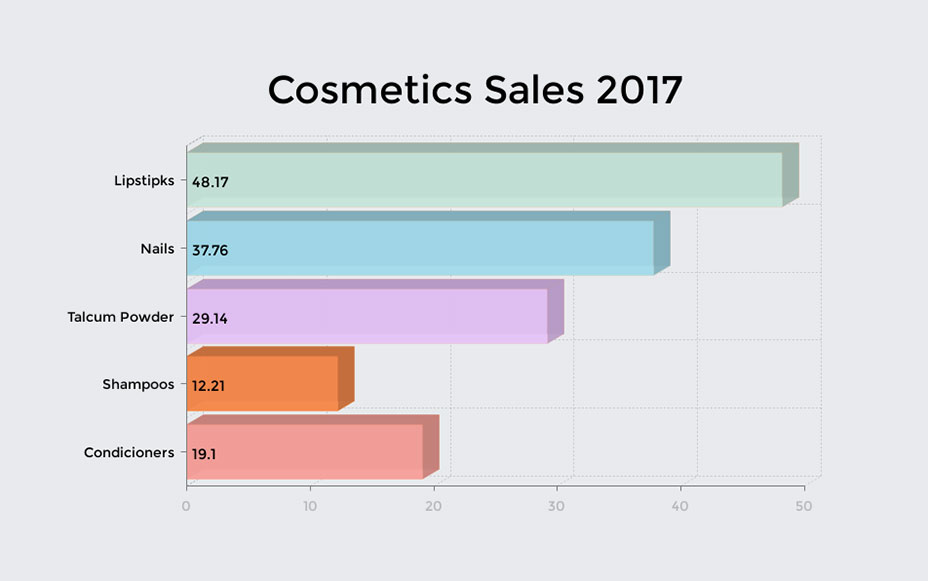Bar graphs, when combined with double and triple charts, are also of benefit in statistical studies as they provide learners with a more defined picture of the dataset by just using simple rectangular bars.

Pictographs, Bar Graphs, and Pie Charts
Pictographs, Bar Graphs, and Pie Charts
Bar Graphs and Pie Charts